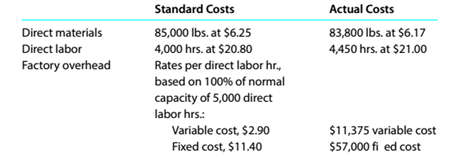Chapter 13, Problem 13.5P

Chapter
Section
Textbook Problem

Direct materials and direct labor, variance analysis; factory overhead cost variance analysis Route 66 Tire Co. manufactures automobile tires. Standard costs and actual costs for direct materials, direct labor, and factory overhead incurred for the manufacture of 10.000 tires were as follows:InstructionsDetermine (a) the price variance, quantity variance, and total direct materials cost variance; (b) the rale variance, time variance, and total direct labor cost variance; and (c) Appendix: variable factory overhead controllable variance, the fixed factory overhead volume variance, and total factory overhead cost variance.

To determine

(a)

Concept Introduction:

Price variance:-It is the difference between price per unit in standard and actual price of product and multiplying that with quantity purchased in actual.

Quantity variance:-It is referred to the amount which is computed by multiplying the standard price per unit with the difference between quantity in actual term and standard term of product.

Direct Material cost variance:-This amount is calculated as the difference between standard cost and actual cost of direct material. The result is favorable when price variance is more than quantity variance. The result is unfavorable when price variance is less than quantity variance.

The price variance, quantity variance and total direct materials cost variance.

Explanation

Computation of Direct material price variance is as follows:

Direct material price variance =(Actual priceStandard price)×Actual quantity=($6.17$6.25)×83,800=\$6,704 Favorable

Computation of Direct material quantity variance is as follows:

Direct material quantity variance =(Actual quantityStandard quantity)<

To determine

(b)

Concept Introduction:

Rate variance:

It is referred to the amount which is computed by multiplying the number of actual hours with the difference between actual rate and standard rate per hour of direct labour.

Time variance:-It is referred to the amount which is computed by multiplying the standard rate per hours with the difference between the number of actual hours and standard hours of direct labour.

Direct labour cost variance:

This amount is calculated as the difference between the actual cost and standard cost of direct labour for production. If answer is in negative than it is favourable. If answer is in positive than it is unfavorable.

The rate variance, time variance and total direct labour cost variance.

To determine

(c)

Concept Introduction:

Volume variance:-It is referred to the amount which is computed by multiplying the fixed standard cost rate per hour with the difference between the actual hours and standard hours of variable factory overhead.

Variable factory overhead cost variance:-This amount is calculated as the difference between the contrallable variance and volume variance of variable factory overhead. If price is in negative, then it is favourable. If price is in positive then it is unfavorable.

The variable factory overhead controlled variance, fixed factory overhead volume variance and total factory overhead cost variance.

Still sussing out bartleby?

Check out a sample textbook solution.

See a sample solution

The Solution to Your Study Problems

Bartleby provides explanations to thousands of textbook problems written by our experts, many with advanced degrees!

Get Started

What is businesses response to environmental concerns?

Foundations of Business (MindTap Course List)

What is the difference between a stock dividend and a stock split? As a stockholder, would you prefer to see yo...

Fundamentals of Financial Management, Concise Edition (with Thomson ONE - Business School Edition, 1 term (6 months) Printed Access Card) (MindTap Course List)

Who are some of the basic users of financial statements, and how do they use them?

Fundamentals of Financial Management, Concise Edition (MindTap Course List)

What is a flexible manufacturing system?

Cornerstones of Cost Management (Cornerstones Series)